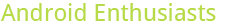## most voted posts

0

(click on this box to dismiss)Q&A for enthusiasts and power users of the Android operating system

``````select
sum(case when votetypeid=1 then 1 else 0 end) as AcceptedByOriginator,
sum(case when votetypeid=2 then 1 else 0 end) as UpMod,
sum(case when votetypeid=3 then 1 else 0 end) as DownMod,
sum(case when votetypeid=4 then 1 else 0 end) as Offensive,
sum(case when votetypeid=5 then 1 else 0 end) as Favorite,
sum(case when votetypeid=6 then 1 else 0 end) as [Close],
sum(case when votetypeid=7 then 1 else 0 end) as Reopen,
sum(case when votetypeid=8 then 1 else 0 end) as BountyStart,
sum(case when votetypeid=9 then 1 else 0 end) as BountyClose,
sum(case when votetypeid=10 then 1 else 0 end) as Deletion,
sum(case when votetypeid=11 then 1 else 0 end) as Undeletion,
sum(case when votetypeid=12 then 1 else 0 end) as Spam,
sum(case when votetypeid=15 then 1 else 0 end) as ModeratorReview,
sum(case when votetypeid=16 then 1 else 0 end) as ApproveEditSuggestionHold tight while we fetch your results